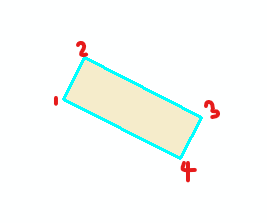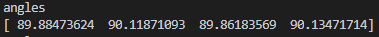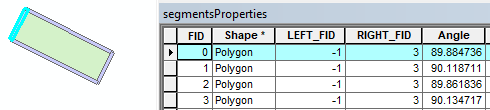# polygon vertex angle arcpy

2188
9
02-21-2020 08:34 AMNew Contributor II

The goal is to find the angle at each vertex. My current computation which started with the clue I got here, isn't giving me the expected vertex angle values. By expected values, I mean angles at vertex measured using ArcMap10 COGO tool.

inFC (input feature class) are polylines which were a result of polygon split operation.

I am also not getting it right with regards to pushing those angle values to the field "Angle"

Alternate approaches are welcome.

``````# get the vertex angle
def getVertexAngle(inFC):

pointer = 0

with arcpy.da.UpdateCursor(inFC, 'Angle') as uCur:
with arcpy.da.SearchCursor(inFC, 'SHAPE@') as sCur:
for row in sCur:
orient = []

Geom = row
Parts = range(Geom.partCount)

for part in Parts:
currPart = Geom.getPart(part)
pointCount = currPart.count

qPointsRange = range(1, pointCount - 1)

for point in qPointsRange:
currPnt = currPart.getObject(point)
X = currPnt.X
Y = currPnt.Y

prevPnt = currPart.getObject(point - 1)
nxtPnt = currPart.getObject(point + 1)

radian = math.atan2(Y - prevPnt.Y, X - prevPnt.X) - math.atan2(nxtPnt.Y - prevPnt.Y, nxtPnt.X - prevPnt.X)

orient.append(degrees)

print degrees

print "orientations are: ", orient
return orient‍‍‍‍‍‍‍‍‍‍‍‍‍‍‍‍‍‍‍‍‍‍‍‍‍‍‍‍‍‍‍‍‍‍‍‍‍‍``````
1 Solution

Accepted SolutionsbyMVP Legendary Contributor

This will return either interior or exterior angles.

I didn't know what you want to do with the list, so this example simply prints the list of interior angles for a featureclass with 6 polygons.

``````import numpy as np
import arcpy

def _poly_arr_(poly):
"""Return coordinates of nested objects."""
def _split_(part):
yield [(p.X, p.Y) for p in part if p]
# ----
arrs =[]
for part in poly:
out = []
w = np.where(np.isin(part, None, invert=False))
bits = np.split(part, w)
for i in bits:
sub = _split_(i)
out.append(np.array(*sub).squeeze())
arrs.append(np.asarray(out).squeeze())
return np.asarray(arrs)

def _angles_(a, inside=True, in_deg=True):
"""Worker for Geo `polygon_angles` and `polyline_angles`.

Parameters
----------
inside : boolean
True, for interior angles.
in_deg : boolean
True for degrees, False for radians.
"""
# ----
def _x_(a):
"""Cross product.  see npg_helpers as well."""
ba = a - np.concatenate((a[-1, None], a[:-1]), axis=0)
bc = a - np.concatenate((a[1:], a[0, None]), axis=0)
return np.cross(ba, bc), ba, bc
# ----
if np.allclose(a, a[-1]):                 # closed loop, remove dupl.
a = a[:-1]
cr, ba, bc = _x_(a)
dt = np.einsum('ij,ij->i', ba, bc)
ang = np.arctan2(cr, dt)
TwoPI = np.pi * 2.
if inside:
angles = np.where(ang < 0, ang + TwoPI, ang)
else:
angles = np.where(ang > 0, TwoPI - ang, ang)
if in_deg:
angles = np.degrees(angles)
return angles

# ----- Change the line below *******************************
in_fc = r"C:\Folder_to_your\shapefile.shp"  # ---- not tested

with arcpy.da.SearchCursor(in_fc, "SHAPE@") as cur:
for row in cur:
geom = row
arr = _poly_arr_(geom).squeeze()
ang = _angles_(arr, inside=True, in_deg=True)
print("angles\n{}".format(ang))

‍‍‍‍‍‍‍‍‍‍‍‍‍‍‍‍‍‍‍‍‍‍‍‍‍‍‍‍‍‍‍‍‍‍‍‍‍‍‍‍‍‍‍‍‍‍‍‍‍‍‍‍‍‍‍‍‍‍‍‍‍‍‍‍‍‍‍‍‍‍‍‍‍‍‍‍‍‍‍‍‍‍‍‍‍‍‍‍‍‍‍‍‍‍‍‍‍‍‍‍‍‍‍‍‍‍‍‍‍‍‍‍‍‍‍‍‍‍‍‍‍‍‍‍‍‍‍``````

Results

``````angles
[90. 90. 90. 90.]
angles
[ 36.86989765  63.43494882  34.69515353 225.        ]
angles
[ 34.69515353 225.          36.86989765  63.43494882]
angles
[63.43494882 53.13010235 63.43494882]
angles
[ 90. 270.  90.  90. 270.  90.  90. 270.  90.  90. 270.  90.]
angles
[ 90.  90.  90.  90. 270.  90.]‍‍‍‍‍‍‍‍‍‍‍‍``````

The featureclass9 RepliesbyMVP

I came across a post that sounded similar to your needs and it looked promising.

Hope this helpsNew Contributor II

Thank you for this Jeremy. This indeed computes vertex angle but I was looking for a solution for a closed geometry (Polygon) as this is the major issue for me.byMVP Legendary Contributor

This will return either interior or exterior angles.

I didn't know what you want to do with the list, so this example simply prints the list of interior angles for a featureclass with 6 polygons.

``````import numpy as np
import arcpy

def _poly_arr_(poly):
"""Return coordinates of nested objects."""
def _split_(part):
yield [(p.X, p.Y) for p in part if p]
# ----
arrs =[]
for part in poly:
out = []
w = np.where(np.isin(part, None, invert=False))
bits = np.split(part, w)
for i in bits:
sub = _split_(i)
out.append(np.array(*sub).squeeze())
arrs.append(np.asarray(out).squeeze())
return np.asarray(arrs)

def _angles_(a, inside=True, in_deg=True):
"""Worker for Geo `polygon_angles` and `polyline_angles`.

Parameters
----------
inside : boolean
True, for interior angles.
in_deg : boolean
True for degrees, False for radians.
"""
# ----
def _x_(a):
"""Cross product.  see npg_helpers as well."""
ba = a - np.concatenate((a[-1, None], a[:-1]), axis=0)
bc = a - np.concatenate((a[1:], a[0, None]), axis=0)
return np.cross(ba, bc), ba, bc
# ----
if np.allclose(a, a[-1]):                 # closed loop, remove dupl.
a = a[:-1]
cr, ba, bc = _x_(a)
dt = np.einsum('ij,ij->i', ba, bc)
ang = np.arctan2(cr, dt)
TwoPI = np.pi * 2.
if inside:
angles = np.where(ang < 0, ang + TwoPI, ang)
else:
angles = np.where(ang > 0, TwoPI - ang, ang)
if in_deg:
angles = np.degrees(angles)
return angles

# ----- Change the line below *******************************
in_fc = r"C:\Folder_to_your\shapefile.shp"  # ---- not tested

with arcpy.da.SearchCursor(in_fc, "SHAPE@") as cur:
for row in cur:
geom = row
arr = _poly_arr_(geom).squeeze()
ang = _angles_(arr, inside=True, in_deg=True)
print("angles\n{}".format(ang))

‍‍‍‍‍‍‍‍‍‍‍‍‍‍‍‍‍‍‍‍‍‍‍‍‍‍‍‍‍‍‍‍‍‍‍‍‍‍‍‍‍‍‍‍‍‍‍‍‍‍‍‍‍‍‍‍‍‍‍‍‍‍‍‍‍‍‍‍‍‍‍‍‍‍‍‍‍‍‍‍‍‍‍‍‍‍‍‍‍‍‍‍‍‍‍‍‍‍‍‍‍‍‍‍‍‍‍‍‍‍‍‍‍‍‍‍‍‍‍‍‍‍‍‍‍‍‍``````

Results

``````angles
[90. 90. 90. 90.]
angles
[ 36.86989765  63.43494882  34.69515353 225.        ]
angles
[ 34.69515353 225.          36.86989765  63.43494882]
angles
[63.43494882 53.13010235 63.43494882]
angles
[ 90. 270.  90.  90. 270.  90.  90. 270.  90.  90. 270.  90.]
angles
[ 90.  90.  90.  90. 270.  90.]‍‍‍‍‍‍‍‍‍‍‍‍``````

The featureclassNew Contributor II

Thank you Dan. Currently not on my computer but will try it out. However, how do I work with this with an input shapefile? A bit unclear on how to use.byMVP Legendary Contributor

I edited my original post with a fuller example.New Contributor II

Thank you, Dan. To answer what the purpose is, I want to know the values of each polygon vertex and based on the value of inaccuracies with respect to expected values, I will be moving each side of the polygon (split into segments) to the expected values.

For example, for corners which should be 90°, and I get 89.83°, I will need to rotate the line segment. So, my current question above is just the start step I will be needing.

I will implement your suggestion above and revert once on my computer. Thank you!byMVP Legendary Contributor

Keep us posted.

As an explanation,

• the einsum line does a dot product for all segment points at one
• the crossproduct implementation does a similar thing (_x_)
• The arctan2 simply implements the dy, dx all at once too.

I use numpy when I am working with geometries that may have hundreds or thousands or edges.  It may seem like overkill for simple shapes but the process-by-triplet logic is still there, except it is completely vectorized.  I kept the searchcursor bits if that is way you collect geometry in your workflow.New Contributor II

Thank you, Dan. This did work well in determining the polygon angles as well as polyline angles (arcpy.PolygonToLine_management() ). However, I was wondering how I would get to identify the vertex angle that it had computed.Currently, the above images show how the angles tally with the vertex using the COGO tool to investigate.

Just as a note:

Also, (guessing the numpy version on the machine is pretty old), I had to change line 12 to read as:

``w = np.where(np.is1d(part, None, invert=False))``

I got this hint from here as I was having an "isin" error not found in numpy module.

EDIT 1: Added more details to describe the result.

EDIT 2: Added a more situational result. (New image with attribute table alongside geometry)New Contributor II

Resolved seeing angles were measured clockwise. Thanks to Dan.